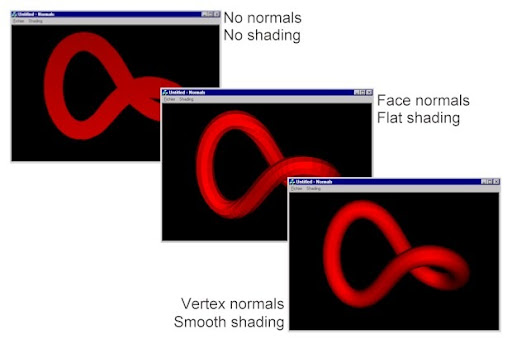## Thursday, April 03, 2008

### Vertex Normals

Recently I had to create a 3D model in OpenFlight. The model was made up of a mesh - a list of points and a list of triangles that reference the points in the list by index.

Writing out the mesh just as it is - leads to a flat colored object.

The reason for this is that without normals - the viewer doesn't know how light is reflected off the faces of the model. This is why we need to provide information about the normals for the model. Normals are used in Gouraud shading, Phong shading and other lighting models.

From all my experience with 3D graphics - there was one normal assigned to each face in the model. In other examples I have worked with - this surface normal is then applied to each vertex that makes up the face - a simplified vertex normal.

The only issue with one normal being assigned to all vertices connected to a face - is that the model will have sharp edges - even though it might be a smooth surface. This can look especially terrible when you try and model cylinders, domes or any surface that has a curve on it.

To alleviate this problem - vertices are assigned normals based on the average of all the normals of the faces that, that vertex is a part of.The above example shows an example of shading based on different types of normals.

From left to right:

• No normals - the first image shows what a 3d model looks like without any normal information - it looks flat.
• Normals based on the main face - when normals are assigned based on just the main face that they belong to - then the model looks very faceted.
• Vertex normals based on a combination of surface normals of the faces that contain that vertex - then the model results in a smooth - realistic looking 3d model.

What you need for vertex normal calculations:

1. Code to compute normal
The easiest way to do this is to treat the edges of the triangle as vectors (where the triangle is a face of the mesh/object).
The normal is then computed as the cross product of 2 of the adjacent vectors.
The normal is then normalized.

As pseudo-code

• for each vertex i in VertexList v
• n <== Zero Vector
• for each triangle j that shares ith vertex
• n <== n + Normalize( Normal(triangle j) )
• end for
• n[i] = Normalize(n)
• end for

An optimization:

If you look at the above pseudo-code - you will notice that the algorithm runs in will take (n x m) loops to complete, where n is number of vertices and m is the number of triangles. Typically m can approach and even exceed the number of vertices n. Thus the above algorithm has a complexity close to O(n2).

A useful optimization that one can use is instead of looping over the vertices - you loop over the triangles.

• for each vertex i in VertexList v
• n[i] <== Zero Vector
• for each triangle j in TriangleList t
• n <== Normalize( Normal(triangle j) )
• for each vertex i in triangle j
• n[i] <== n[i] + n
• end for
• end for
• for each vertex i in VertexList v
• n[i] <== Normalize(n[i])
• end for

This reduces the complexity of the algorithm to O(m) and runs much faster than the original algorithm.

Finally - if you look at the paper Generating Vertex Normals, you will see that there are some useful heuristics that you can apply for more realistic effects based on the application. (such as weighting the average of the normal based on the area of the triangle - vectors make it easy to compute the area of a triangle too).

Useful articles on vertex normal computation:

#### Generating Vertex Normals (pdf - contains pseudocode for vertex normal calculation)

Face and Vertex Normal Vectors (Direct3D 9)

A Vector Type for C# - a vector class with useful methods for performing vector arithmetic.